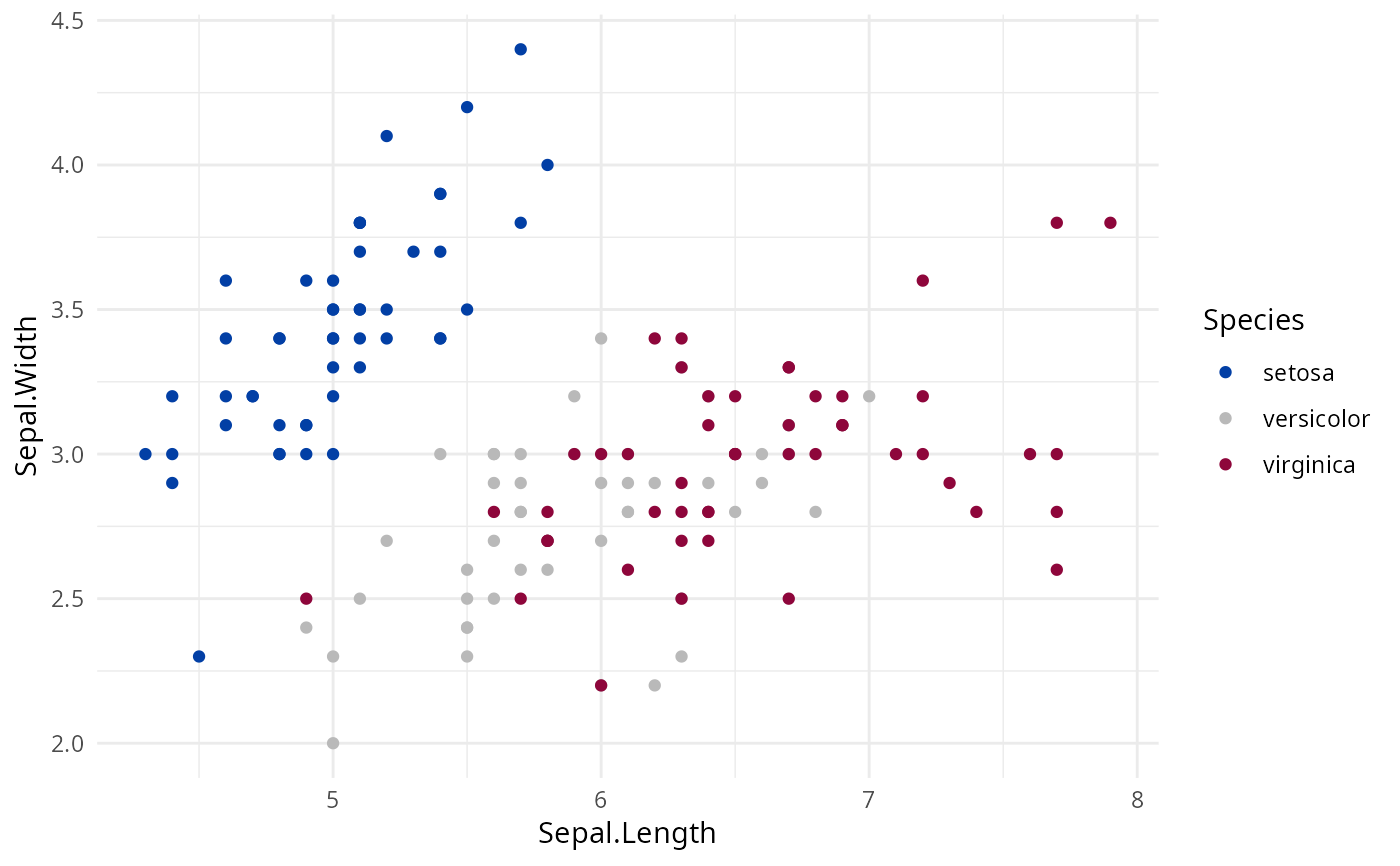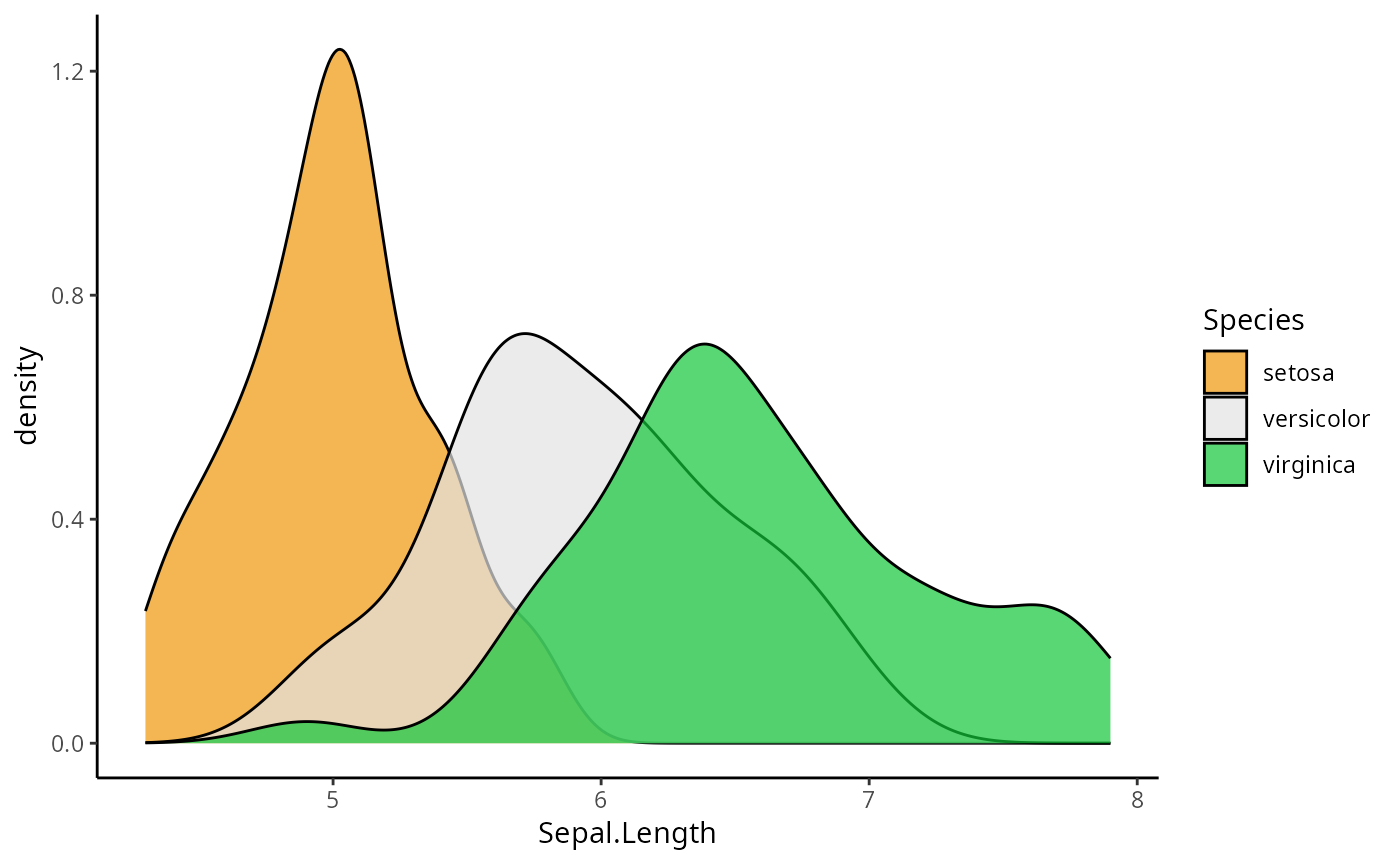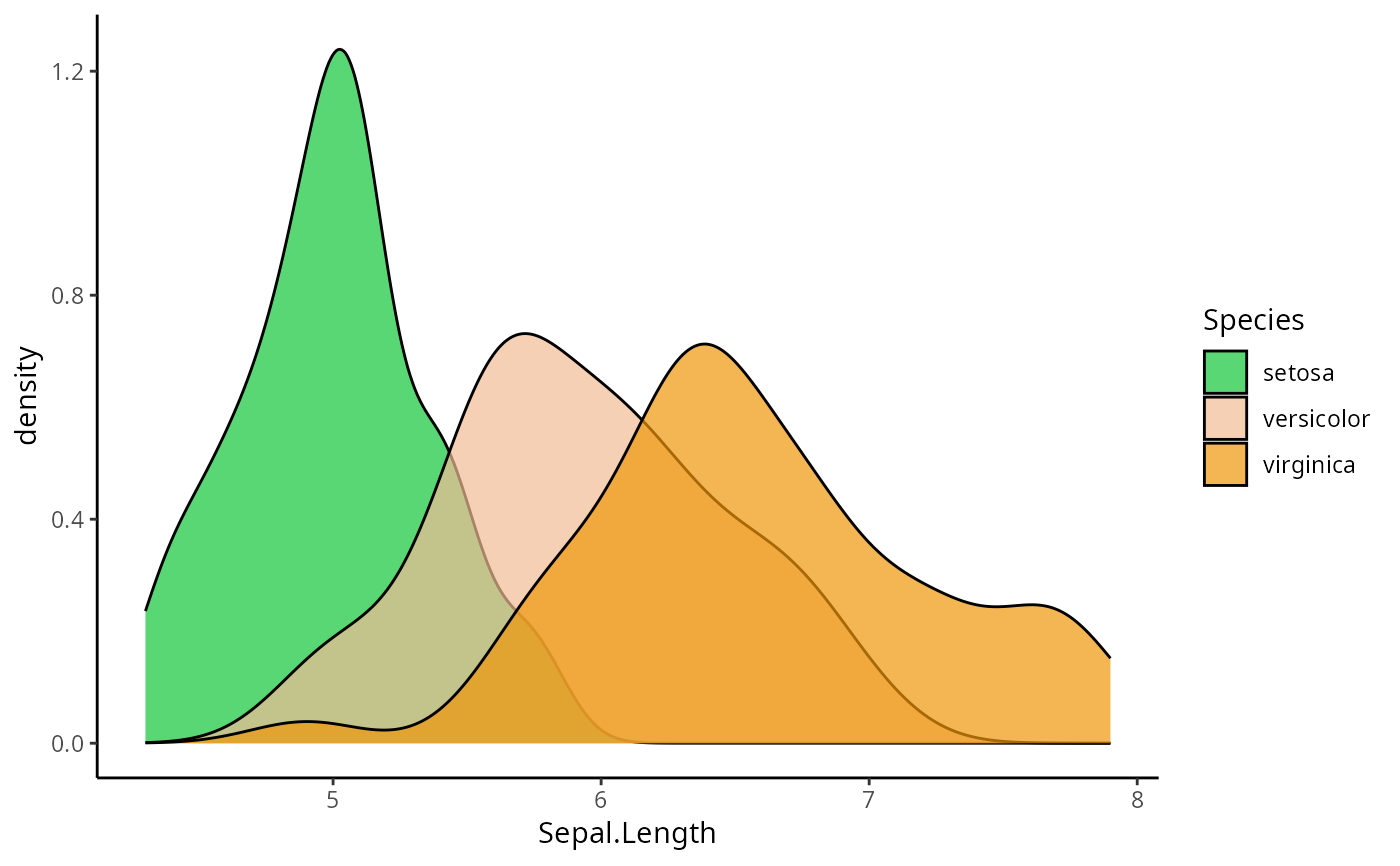Discrete ggplot2 color scales using the color palettes generated by diverging_hcl.

scale_colour_discrete_diverging(
palette = NULL,
c1 = NULL,
cmax = NULL,
l1 = NULL,
l2 = NULL,
h1 = NULL,
h2 = NULL,
p1 = NULL,
p2 = NULL,
alpha = 1,
rev = FALSE,
nmax = NULL,
order = NULL,
aesthetics = "colour",
...
)

scale_color_discrete_diverging(
palette = NULL,
c1 = NULL,
cmax = NULL,
l1 = NULL,
l2 = NULL,
h1 = NULL,
h2 = NULL,
p1 = NULL,
p2 = NULL,
alpha = 1,
rev = FALSE,
nmax = NULL,
order = NULL,
aesthetics = "colour",
...
)

scale_fill_discrete_diverging(..., aesthetics = "fill")

## Arguments

palette

The name of the palette to be used. Run hcl_palettes(type = "diverging") for available options.

c1

Chroma value at the scale endpoints.

cmax

Maximum chroma value.

l1

Luminance value at the scale endpoints.

l2

Luminance value at the scale midpoint.

h1

Hue value at the first endpoint.

h2

Hue value at the second endpoint.

p1

Control parameter determining how chroma should vary (1 = linear, 2 = quadratic, etc.).

p2

Control parameter determining how luminance should vary (1 = linear, 2 = quadratic, etc.).

alpha

Numeric vector of values in the range [0, 1] for alpha transparency channel (0 means transparent and 1 means opaque).

rev

If TRUE, reverses the order of the colors in the color scale.

nmax

Maximum number of different colors the palette should contain. If not provided, is calculated automatically from the data.

order

Numeric vector listing the order in which the colors should be used. Default is 1:nmax.

aesthetics

The ggplot2 aesthetics to which this scale should be applied.

...

common discrete scale parameters: name, breaks, labels, na.value, limits and guide. See discrete_scale for more details.

## Details

If both a valid palette name and palette parameters are provided then the provided palette parameters overwrite the parameters in the named palette. This enables easy customization of named palettes.

## Examples

library("ggplot2")

# default colors with slightly darkened midpoint
ggplot(iris, aes(Sepal.Length, Sepal.Width, color = Species)) +
geom_point() + theme_minimal() +
scale_color_discrete_diverging(l2=75)# color scale "Green-Orange"
ggplot(iris, aes(Sepal.Length, fill = Species)) +
geom_density(alpha = 0.7) + theme_classic() +
scale_fill_discrete_diverging(palette = "Green-Orange", rev = TRUE)# use nmax and order to skip some colors
ggplot(iris, aes(Sepal.Length, fill = Species)) +
geom_density(alpha = 0.7) + theme_classic() +
scale_fill_discrete_diverging(palette = "Green-Orange", nmax = 5, order = c(1, 4, 5))